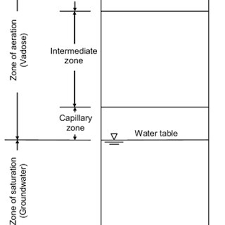## How to Calculate and Solve for Storage Co-efficient for a Confined Aquifer | Water BudgetThe image above represents storage co-efficient for a confined aquifer.

To compute for storage co-efficient for a confined aquifer, five essential parameters are needed and these parameters are Unit Weight of Water (γw), Confined Aquifer Thickness (H), Compressibility of the Aquifer Material (α), Porosity of Aquifer Material (n) and Compressibility of Water (β).

The formula for calculating storage co-efficient for a confined aquifer:

S = γw(α + nβ)H

Where;

S = Storage Co-efficient for a Confined Aquifer
γw = Unit Weight of Water
H = Confined Aquifer Thickness
α = Compressibility of the Aquifer Material
n = Porosity of Aquifer Material
β = Compressibility of Water

Let’s solve an example;
Find the storage co-efficient for a confined aquifer when the unit weight of water is 8, confined aquifer thickness is 12, the compressibility of the aquifer material is 21, the porosity of aquifer material is 18 and the compressibiity of water is 14.

This implies that;

γw = Unit Weight of Water = 8
H = Confined Aquifer Thickness = 12
α = Compressibility of the Aquifer Material = 21
n = Porosity of Aquifer Material = 18
β = Compressibility of Water = 14

S = γw(α + nβ)H
S = 8(21 + 18(14))12
S = 8(21 + 252)12
S = 8(273)12
S = 26208

Therefore, the storage co-efficient for a confined aquifer is 26208.

Calculating the Unit Weight of Water when the Storage Co-efficient for a Confined Aquifer, the Confined Aquifer Thickness, the Compressibility of the Aquifer Material, the Porosity of Aquifer Material and the Compressibility of Water is Given.

γw = S / (a + nβ)H

Where;

γw = Unit Weight of Water
S = Storage Co-efficient for a Confined Aquifer
H = Confined Aquifer Thickness
α = Compressibility of the Aquifer Material
n = Porosity of Aquifer Material
β = Compressibility of Water

Let’s solve an example;
Find the unit weight of water when the storage co-efficient for a confined aquifer is 20, the confined aquifer thickness is 10, the compressibility of the aquifer material is 8, the porosity of aquifer material is 3 and the compressibility of water is 4.

This implies that;

S = Storage Co-efficient for a Confined Aquifer = 20
H = Confined Aquifer Thickness = 10
α = Compressibility of the Aquifer Material = 8
n = Porosity of Aquifer Material = 3
β = Compressibility of Water = 4

γw = S / (a + nβ)H
γw = 20 / (8 + (3 x 4))10
γw = 20 / (8 + 12)10
γw = 20 / (20)10
γw = 20 / 200
γw = 0.1

Therefore, the unit weight of water is 0.1.